# Calculus 3 : Normal Vectors

## Example Questions

### Example Question #61 : Normal Vectors

Determine whether the two vectors,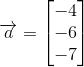and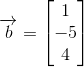, are orthogonal or not.

The two vectors are not orthogonal.

The two vectors are orthogonal.

The two vectors are not orthogonal.

Explanation:

Vectors can be said to be orthogonal, that is to say perpendicular or normal, if their dot product amounts to zero: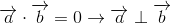To find the dot product of two vectors given the notation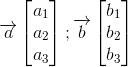Simply multiply terms across rows: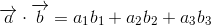For our vectors,and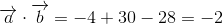The two vectors are not orthogonal.

### Example Question #62 : Normal Vectors

Determine whether the two vectors,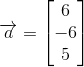and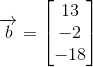, are orthogonal or not.

The two vectors are orthogonal.

The two vectors are not orthogonal.

The two vectors are orthogonal.

Explanation:

Vectors can be said to be orthogonal, that is to say perpendicular or normal, if their dot product amounts to zero:To find the dot product of two vectors given the notationSimply multiply terms across rows:For our vectors,and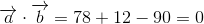The two vectors are orthogonal.

### Example Question #63 : Normal Vectors

Determine whether the two vectors,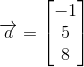and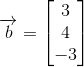, are orthogonal or not.

The two vectors are orthogonal.

The two vectors are not orthogonal.

The two vectors are not orthogonal.

Explanation:

Vectors can be said to be orthogonal, that is to say perpendicular or normal, if their dot product amounts to zero:To find the dot product of two vectors given the notationSimply multiply terms across rows:For our vectors,and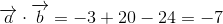The two vectors are not orthogonal.

### Example Question #64 : Normal Vectors

Determine whether the two vectors,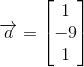and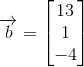, are orthogonal or not.

The two vectors are orthogonal.

The two vectors are not orthogonal.

The two vectors are orthogonal.

Explanation:

Vectors can be said to be orthogonal, that is to say perpendicular or normal, if their dot product amounts to zero:To find the dot product of two vectors given the notationSimply multiply terms across rows:For our vectors,and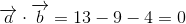The two vectors are orthogonal.

### Example Question #65 : Normal Vectors

Determine whether the two vectors,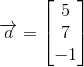and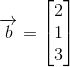, are orthogonal or not.

The two vectors are orthogonal.

The two vectors are not orthogonal.

The two vectors are not orthogonal.

Explanation:

Vectors can be said to be orthogonal, that is to say perpendicular or normal, if their dot product amounts to zero:To find the dot product of two vectors given the notationSimply multiply terms across rows:For our vectors,and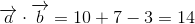The two vectors are not orthogonal.

### Example Question #66 : Normal Vectors

Determine whether the two vectors,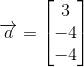and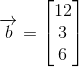, are orthogonal or not.

The two vectors are not orthogonal.

The two vectors are orthogonal.

The two vectors are orthogonal.

Explanation:

Vectors can be said to be orthogonal, that is to say perpendicular or normal, if their dot product amounts to zero:To find the dot product of two vectors given the notationSimply multiply terms across rows:For our vectors,and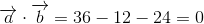The two vectors are orthogonal.

### Example Question #67 : Normal Vectors

Determine whether the two vectors,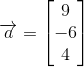and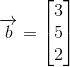, are orthogonal or not.

The two vectors are orthogonal.

The two vectors are not orthogonal.

The two vectors are not orthogonal.

Explanation:

Vectors can be said to be orthogonal, that is to say perpendicular or normal, if their dot product amounts to zero:To find the dot product of two vectors given the notationSimply multiply terms across rows:For our vectors,and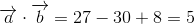The two vectors are not orthogonal.

### Example Question #68 : Normal Vectors

Which of the following vectors is perpendicular to the plane given by the following equation: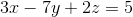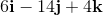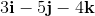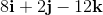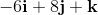Explanation:

A normal vector to a plane of the form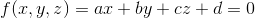is given by the gradient of f.  First, we have to put the equation into a form where it equals zero: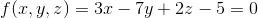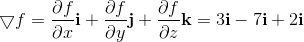A vector multiplied by a constant is parallel to the original vector, so the above vector multiplied by a constant is perpendicular to the plane.  The correct answer is the above vector multiplied by two.

### Example Question #69 : Normal Vectors

Find the tangent vector for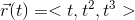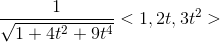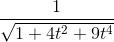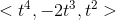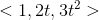Explanation: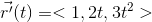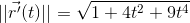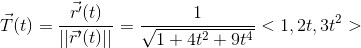### Example Question #70 : Normal Vectors

Find the normal vector (in standard notation) to the plane: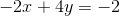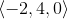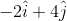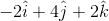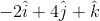Explanation:

To determine the normal vector to a plane, we simply report the coefficients of the x, y, and z terms, as the equation of a plane is given by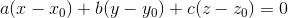where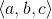is the normal vector.

So, our normal vector isWe were asked to write this in standard notation, which gives us